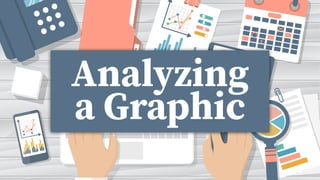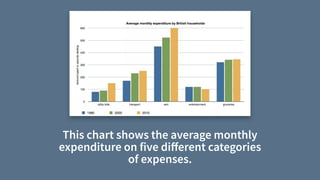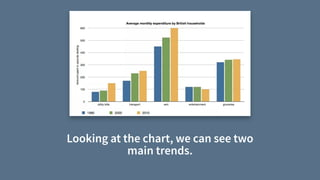Successfully reported this slideshow.

# Analyzing a Graphic.pdf×

# Analyzing a Graphic.pdf

In this presentation, we go through the process of analyzing and describing a bar chart in detail. We look at identifying trends and giving examples that support the trend.

We then look at identifying a second trend in the same chart and describing that one.

In this presentation, we go through the process of analyzing and describing a bar chart in detail. We look at identifying trends and giving examples that support the trend.

We then look at identifying a second trend in the same chart and describing that one.

### Analyzing a Graphic.pdf

1. 1. I Analyzing a Graphic
2. 2. This chart shows the average monthly expenditure on five di ff erent categories of expenses.
3. 3. Looking at the chart, we can see two main trends.
4. 4. 1. Rent was the highest expense throughout the reporting period.
5. 5. 2. Overall, expenditure increased signi fi cantly during the period.
6. 6. Let’s look at each of them…
7. 7. Rent was the highest expense 1
8. 8. First, we need a topic sentence.
9. 9. As shown, rent was the highest expense throughout the period.
10. 10. Next, we need to provide sentences that support this topic.
11. 11. We should start by giving specific information about how much was spent on rent.
12. 12. In 1990, for example, rent was around £450 a month and by 2010 the amount spent on rent reached £600.
13. 13. Then, we need to compare rent with other expenses.
15. 15. By comparison, the amount spent on groceries (the second highest expense) went from £300 in 1990 and £350 in 2010.
16. 16. Now, let’s talk about the other categories.
17. 17. While the amount spent on utility bills and transport also increased, they had only reached £150 and £250, respectively by 2010. The amount spent on entertainment never exceeded more that £110.
18. 18. Finally, we conclude by restating the main point.
19. 19. In conclusion, while most household expenses increased between 1990 and 2010, the amount spent on rent far exceeded the amount spent on anything else.
20. 20. Now, let’s look at the other theme…
21. 21. Overall expenditure increased 1